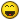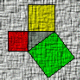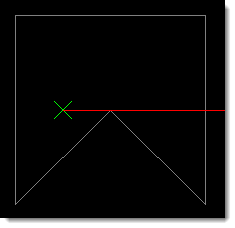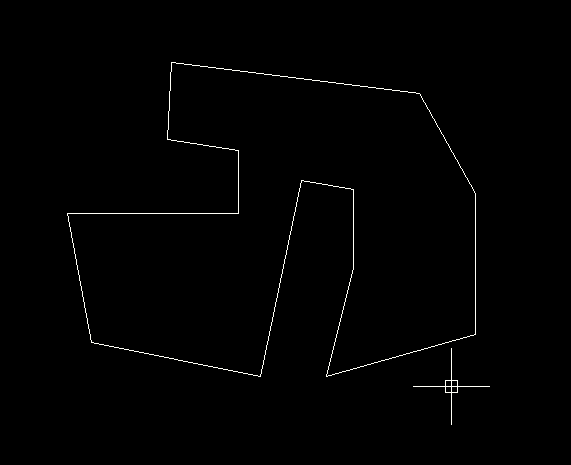# Check if a point is inside an area bounded by a polyline

## Recommended PostsHello!

Is there a mathematical method that can check if a point is inside an area bounded by a polyline?

Graphic method by reporting point P (x, y, z) and then use ssget function to see if it is inside, is slower.

Edited by Costinbos77

##### Share on other sitesI wanted to research this one a while ago, and there is quite a lot of information out there on this question.

Try google searches. I quickly found a thread at the swamp which may help. http://www.theswamp.org/index.php?topic=1890.0 But I think you'd even find this on Wikipedia.The most popular method seems to be send out a few rays at random angles and count the number of intersections. If none of the rays report an even number of intersections with the polygon then it has to lie inside. If I find any more links I'll post them.

##### Share on other sitesCostimbos,

Have a look at this link: Efficient 2-D Geometric Operations by Carlos Moreno.

ymg

##### Share on other sitesHere is a simple function implementing a ray-casting algorithm.

However, note that this function does not test whether the ray 'grazes' a vertex - for example, in the following image the function will incorrectly determine the point as being outside the polyline since the ray intersects the boundary an even number of times.##### Share on other sitesThanks guys, it seems to be a common problem.

##### Share on other sitesMy offer...```(defun c:test ( / poly pt Lv n)
(if (and
(setq poly (car (entsel "\nSelect a Polygon")))
(setq pt (getpoint "\nSelect a point"))
)
(progn
(repeat (setq n (1+ (fix (vlax-curve-getEndParam poly))))
(setq Lv (cons (vlax-curve-getPointAtParam poly (setq n (1- n))) Lv))
)
)
)
)

(defun inside_p (list_vert  / out_p cross on)
(setq out_p (list (car (getvar "extmax")) (* 1.1 (cadr (getvar "extmax")))))
(setq cross 0)
(mapcar
'(lambda (a b)
(if (or
(equal (angle a ) (angle  b) 1e-
(equal a  1e-
)
(setq on t)
)
(if (setq : (inters  out_p a b)) (setq cross (1+ cross)))
)
list_vert
(cdr list_vert)
)
(cond
( on "ON" )
(  (> (rem cross 2) 0) "INSIDE" )
( t "OUTSIDE" )
)
)

```Edited by GP_
Updated code.

##### Share on other sitesThanks GP_ look good.

##### Share on other sitesHi everyone.

I does find GP's routine named Inside_p on this post, but I think it's not complet. I searched for more info on net and find complet code on other post.

```(defun inside_p (list_vert :p / out_p cross on)
(setq out_p (list (car (getvar "extmax")) (* 1.1 (cadr (getvar "extmax")))))
(setq cross 0)
(mapcar
'(lambda (a b)
(if (or
(equal (angle a :p) (angle :p b) 1e-8)
(equal a :p 1e-8)
)
(setq on t)
)
(if (setq :p: (inters :p out_p a b))
(setq cross (1+ cross))
)
)
list_vert
(cdr list_vert)
)
(cond
(on "ON")
((> (rem cross 2) 0) "INSIDE")
(t "OUTSIDE")
)
)```

It's great! but I find a error on use and I don't understand the causes... When I use your code on a USC and north orientation run correctly, but, when i change the plant view, with a Universal system coordinates too, do not work correctly and every time give me Outside... Maybe the extmax variable?

Apologies for my english...

##### Share on other sites```list_vert = Global WCS ,
and  ```
```(getvar "extmax") = Local UCS
```

should be in the same Coordinates System.

you need to convert them with (trans p 0 1)

##### Share on other sitesYou are right...

Thank you Costinbos77

## Join the conversation

You can post now and register later. If you have an account, sign in now to post with your account.
Note: Your post will require moderator approval before it will be visible.×   Pasted as rich text.   Paste as plain text instead

Only 75 emoji are allowed.